Question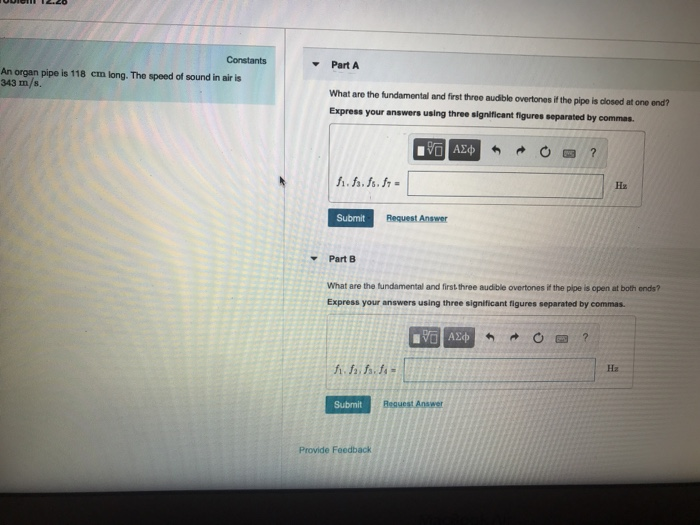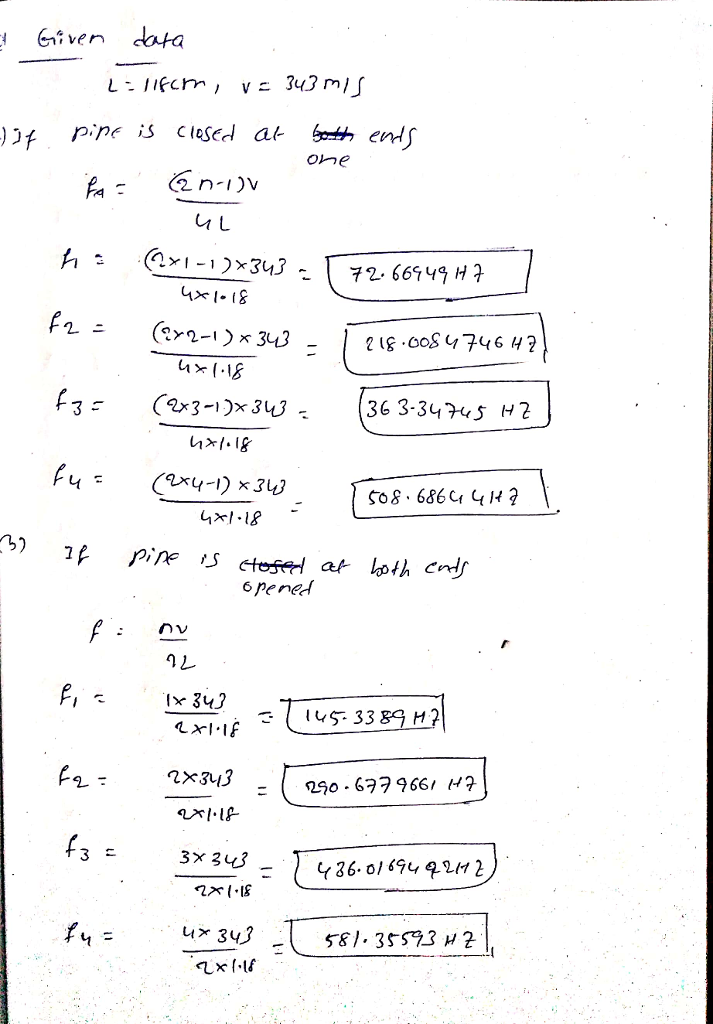#### Earn Coins

Coins can be redeemed for fabulous gifts.

Similar Homework Help Questions
• ### An organ pipe is 109 cm long. Take the speed of sound in ar to be...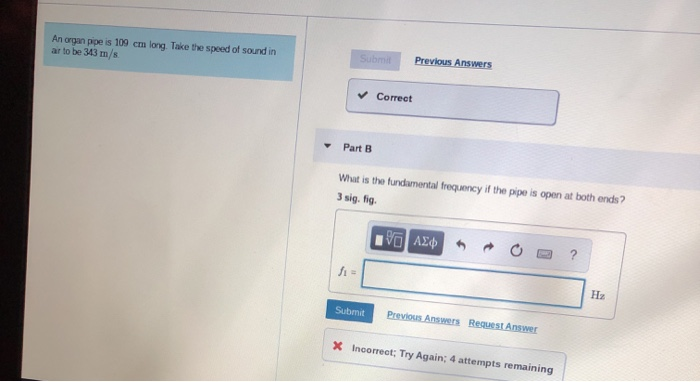An organ pipe is 109 cm long. Take the speed of sound in ar to be 343 m/s Submit Previous Answers Correct Part B What is the fundamental frequency if the pipe is open at both ends? 3 sig. fig. VAEV O ? H2 Submit Previous Answers Request Answer X Incorrect; Try Again: 4 attempts remaining

• ### Waves and Optics Problem 12.21 9 of 18 If the pipe is open at both ends,...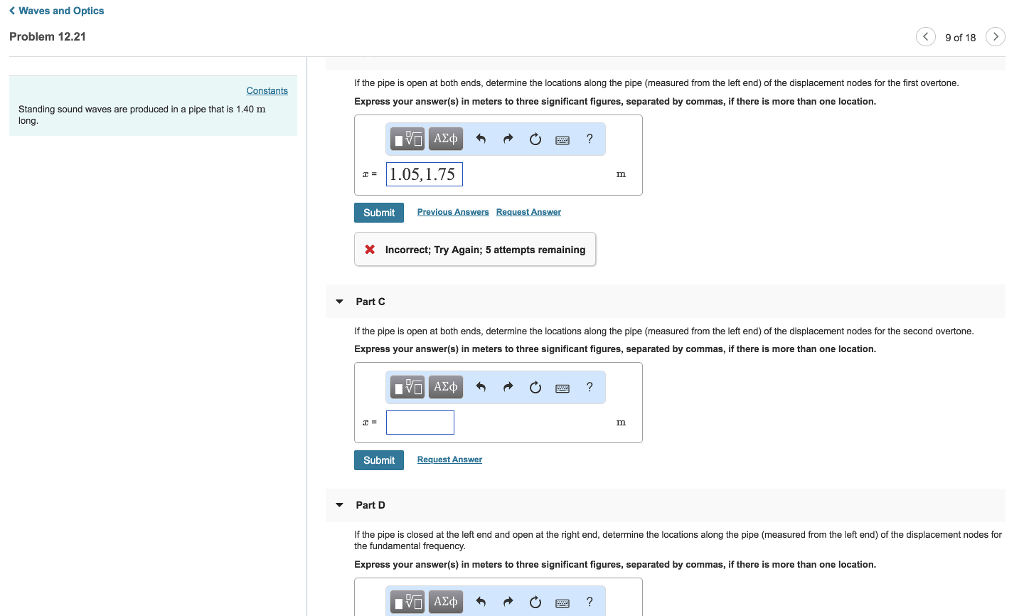Waves and Optics Problem 12.21 9 of 18 If the pipe is open at both ends, determine the locations along the pipe (measured from the left end) of the displacement nodes for the first overtone. Constants Express your answer(s) in meters to three significant figures, separated by commas, if there is more than one location. Standing sound waves are produced in a pipe that is 1.40 m long 1.05,1.75 Submit Previous Answers Reguest Answer X Incorrect; Try Again; 5 attempts...

• ### An organ pipe is 120 cm long. What are the fundamental and first three audible overtones...

An organ pipe is 120 cm long. What are the fundamental and first three audible overtones if the pipe is (a) closed at one end, and (b) open at both ends? 1st overtone(2nd harmonic) if closed at one end? Fundamental(1st harmonic) if closed at one end? Fundamental(1st harmonic) if open at both ends? 2nd overtone(5th harmonic) if closed at one end? 2nd overtone(4th harmonic) if closed at one end? 1st overtone(2nd harmonic) if open at both ends? 3rd overtone(7th harmonic)...

• ### Part A At T = 18 ∘C, how long must an open organ pipe be to...

Part A At T = 18 ∘C, how long must an open organ pipe be to have a fundamental frequency of 349 Hz ? The speed of sound in air is v≈(331+0.60T)m/s, where T is the temperature in ∘C. Express your answer to three significant figures and include the appropriate units. l l = nothingnothing SubmitRequest Answer Part B If this pipe is filled with helium at 20∘C and 1 atm, what is its fundamental frequency? The speed of sound...

• ### Standing sound waves are produced in a pipe that is 1.20 m long. a)If the pipe...

Standing sound waves are produced in a pipe that is 1.20 m long. a)If the pipe is closed at the left end and open at the right end, determine the locations along the pipe (measured from the left end) of the displacement nodes for the first overtone. Express your answer(s) in meters to three significant figures, separated by commas, if there is more than one location. b)If the pipe is closed at the left end and open at the right...

• ### Problem 15.50 Constants I Periodic Table Part A A guitar string is 90.0 cm long and...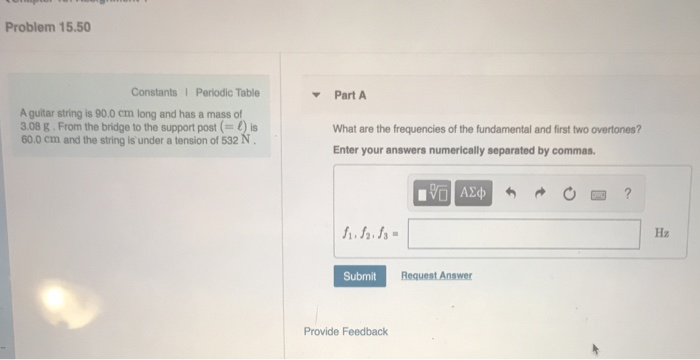Problem 15.50 Constants I Periodic Table Part A A guitar string is 90.0 cm long and has a mass of 3.08 g. From the bridge to the support post () is 60.0 cm and the string is' under a tension of 532 N What are the frequencies of the fundamental and first two overtones? Enter your answers numerically separated by commas. ν ΑΣφ 5 Hz Request Answer Submit Provide Feedback

• ### Constants Part A Suppose that you are in a 15-m-long hallway with all doors closed. Model...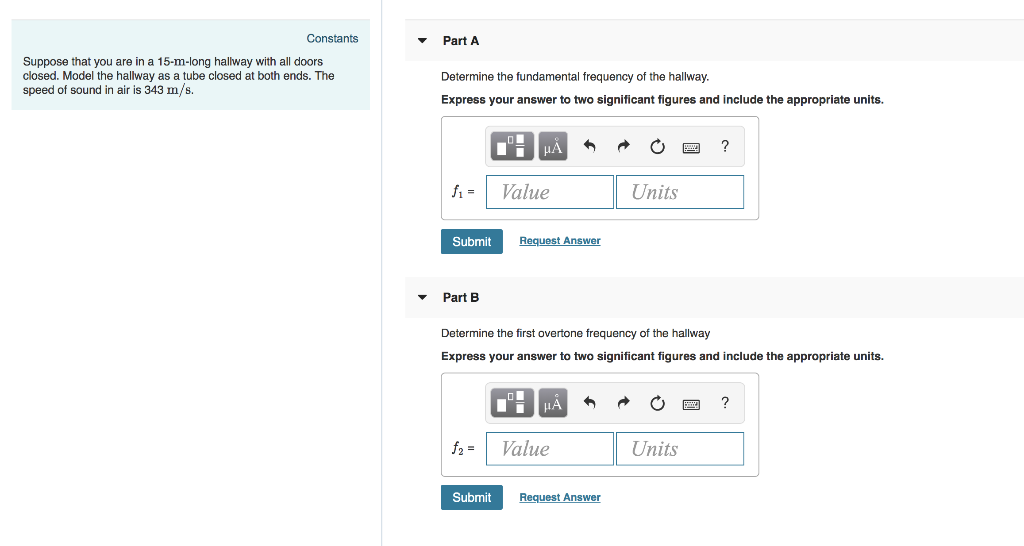Constants Part A Suppose that you are in a 15-m-long hallway with all doors closed. Model the hallway as a tube closed at both ends. The speed of sound in air is 343 m/s. Determine the fundamental frequency of the hallway Express your answer to two significant figures and include the appropriate units. Value Units Submit Request Answer Part B Determine the first overtone frequency of the hallway Express your answer to two significant figures and include the appropriate units....

• ### Constants Part A A gutar string is 90 cm long and has a mass of 38...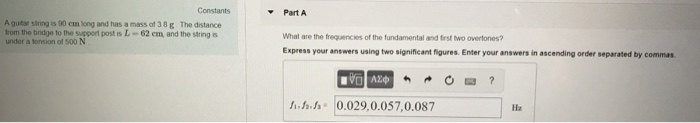Constants Part A A gutar string is 90 cm long and has a mass of 38 g The distance rom the bidge to the support post is L- 62 em, and the string under a tonsion of 500 N What are the frequencies of the fundamental and irst two overtones? Express your answers using two significant figures. Enter your answers in ascending order separated by commas んふか(0.029,0.057,0.087

• ### PartA Constants What is the frequency of its fundamental mode of vibration? Express your answer in...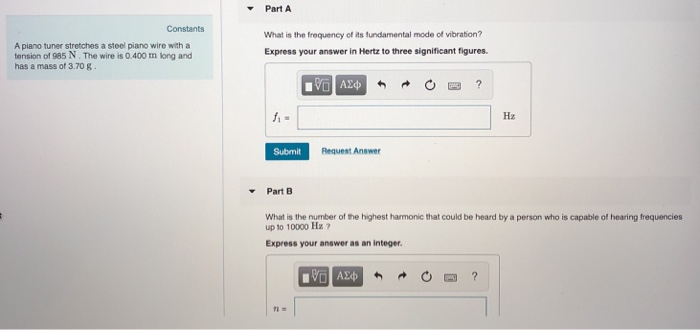PartA Constants What is the frequency of its fundamental mode of vibration? Express your answer in Hertz to three significant figures. A piano tuner stretches a steel piano wire with a tension of 985 N. The wire is 0.400 m long and has a mass of 3.70 B Hz Submit Request Answer PartEB What is the number of the highest harmonic that could be beard by a person who is capable of hearing frequencies up to 10000 Hz ? Express...

• ### Homework 11 Problem 5.76 5 of 7> ▼PartA The member is supported by a pin at...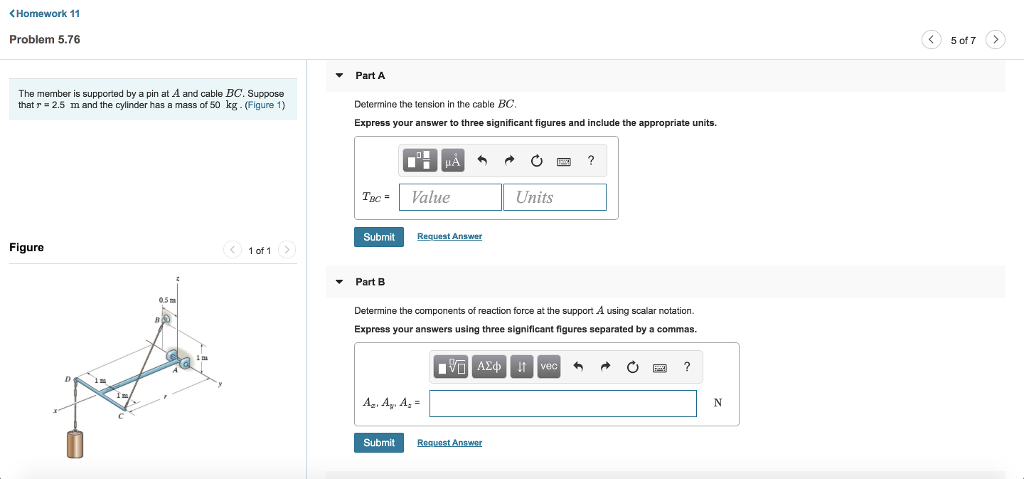Homework 11 Problem 5.76 5 of 7> ▼PartA The member is supported by a pin at A and cable BC. Suppose that r = 2.5 m and the cylinder has a mass of 50 kg. (Figure 1) Determine the tension in the cable BC Express your answer to three significant figures and include the appropriate units. Tac- Value Units Submit Request Answer Figure 1 of 1 ▼ Part B 0.5 Determine the components of reaction force at the support A...